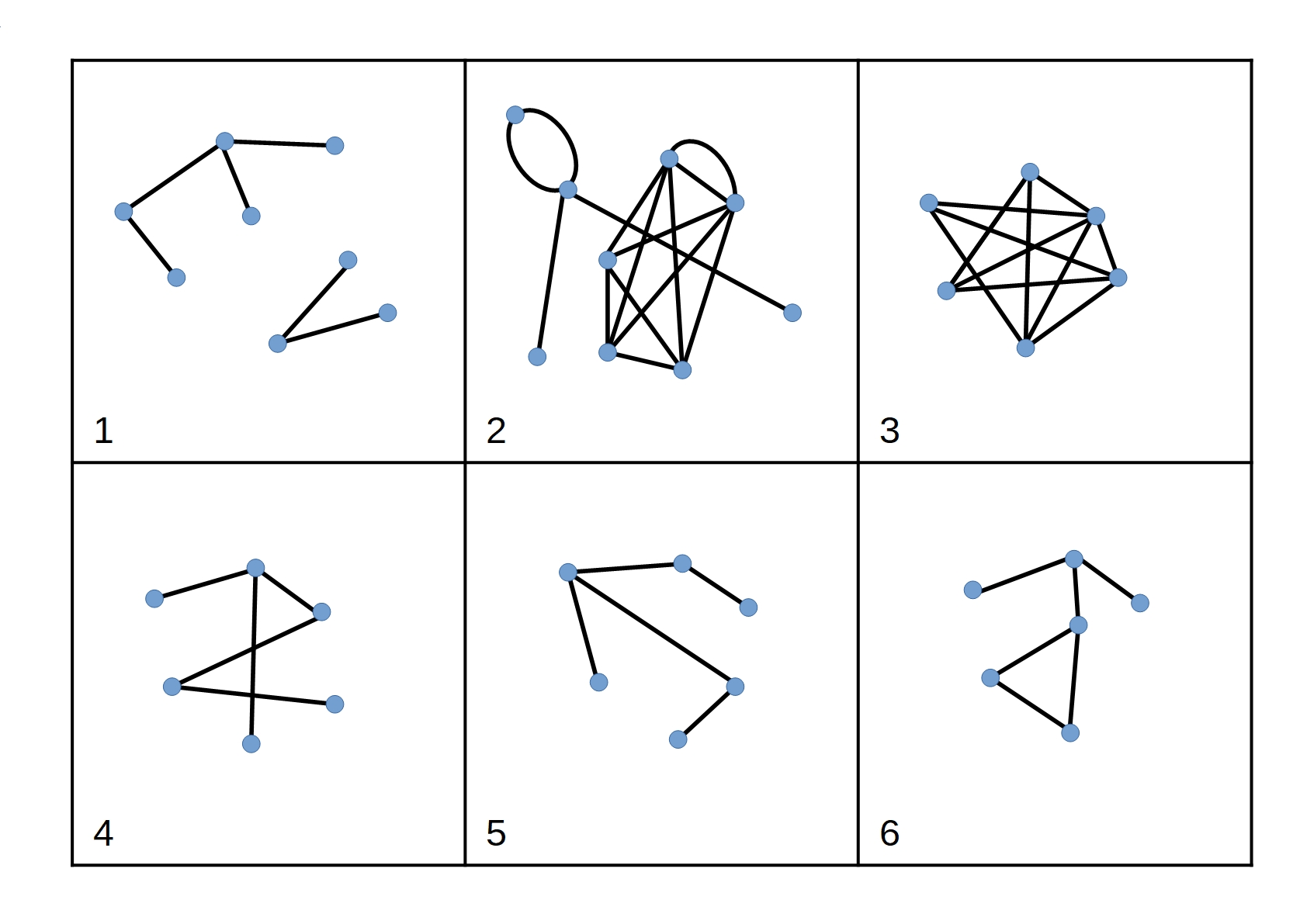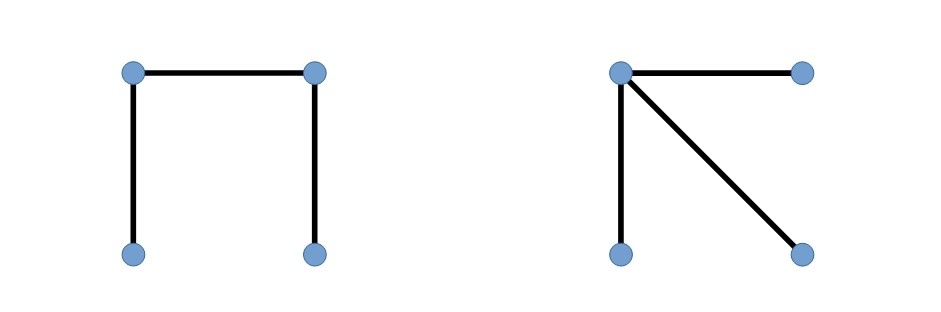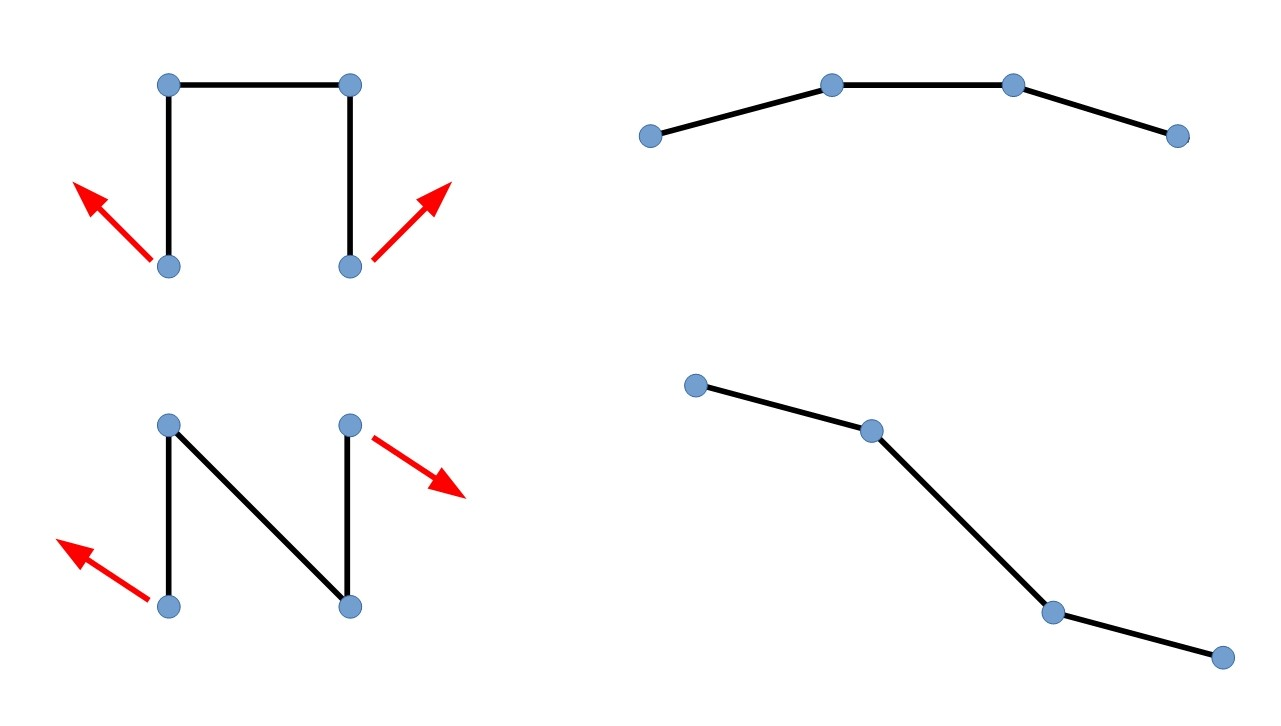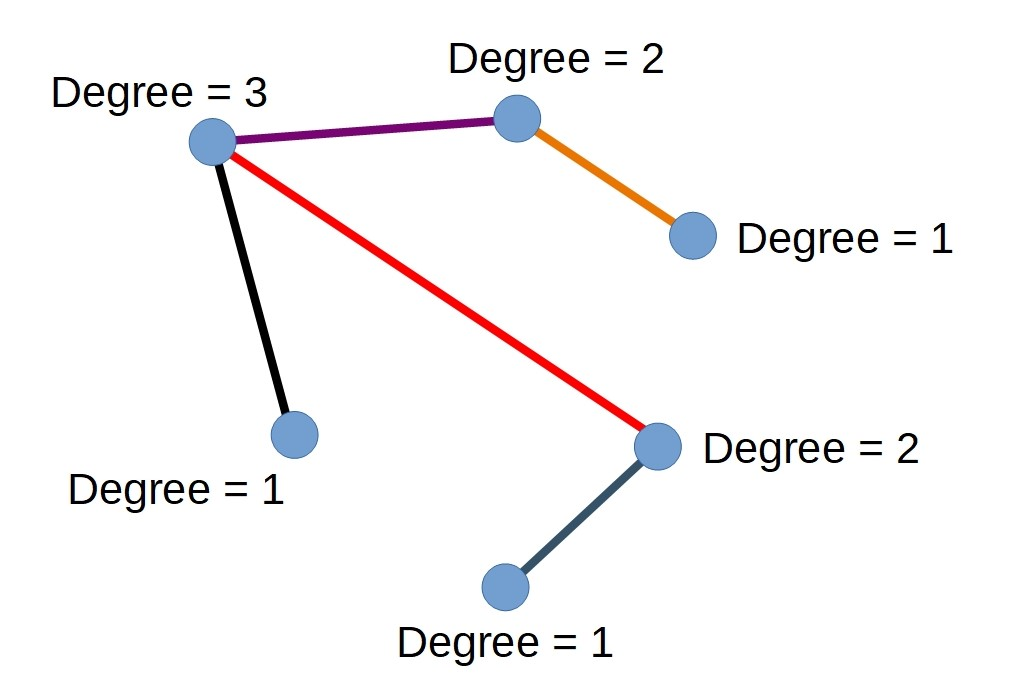#### You may also like### Doodles

Draw a 'doodle' - a closed intersecting curve drawn without taking pencil from paper. What can you prove about the intersections?### Russian Cubes

I want some cubes painted with three blue faces and three red faces. How many different cubes can be painted like that?### Areas and Ratios

Do you have enough information to work out the area of the shaded quadrilateral?

# Network Trees

##### Age 14 to 18Challenge Level

A network is a collection of points and lines between the points. The points are called vertices and the lines which connect them are called edges. Each edge has a vertex at each end.

Here are some possible networks:A connected network is a network in which we can get from any vertex to any other by travelling along the edges.
A planar network is one which can be redrawn in such a way that no edges cross each other.
A cyclic network is one where there is a closed circuit or loop somewhere in the network.  A network with no closed circuits is an acyclic network.

Describe each of the networks above with the words connected/disconnected, planar/non-planar and cyclic/acyclic.

1. Disconnected, Planar, Acyclic
2. Disconnected, Non-planar, Cyclic
3. Connected, Planar, Cyclic
4. Connected, Planar, Acyclic (note that this can be redrawn in such a way that the edges do not cross)
5. Connected, Planar, Acyclic
6. Connected, Planar, Cyclic

Draw a network which is Disconnected, Planar and Cyclic?

Can you draw a network which is Non-planar and Acyclic?

A network is called a tree if it is connected, planar and acyclic (like networks 4 and 5 above).

Investigate the number of edges in trees with different numbers of vertices.
Can you prove a relationship between the number of vertices in a tree and the number of edges?

What happens to the tree when you add an extra edge?
Remember that the network must not be cyclic.

There are two different trees which have 4 vertices.Note that the following two trees do not count as different as we can stretch each of them out as a straight line.How many different trees can you draw with 5 vertices?

There are three different trees with 5 vertices

The degree of a vertex is the number of edges that meet at the vertex.Draw all the possible trees with 2, 3 and 4 vertices.
Label the vertices on your drawings with the degree of each vertex.
Do the same for your trees with 5 vertices.
What can you say about the sum of the degrees of the vertices in trees with 2, 3, 4, 5, ... $n$ vertices?
Can you explain what you have noticed?

How do the number of vertices relate to the number of edges?
How does each edge contribute to the degrees of the vertices?

How many different trees can you draw with 6 vertices?

There are five different ways you can write 10 as the sum of six positive integers:
1, 1, 1, 1, 1, 5
1, 1, 1, 1, 2, 4
1, 1, 1, 1, 3, 3
1, 1, 1, 2, 2, 3
1, 1, 2, 2, 2, 2
One of these combinations produces more than one tree.

How many trees can you draw with 7 vertices?
How can you use your results so far to convince yourself that you have found all of the possible trees?